# Semantics In Text Chapter 3 1 Outline Semantics

• Slides: 38Semantics In Text: Chapter 3 1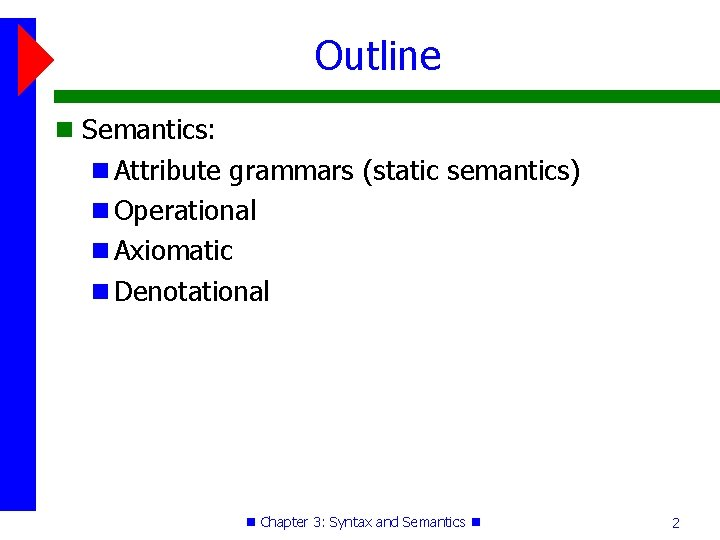Outline Semantics: Attribute grammars (static semantics) Operational Axiomatic Denotational Chapter 3: Syntax and Semantics 2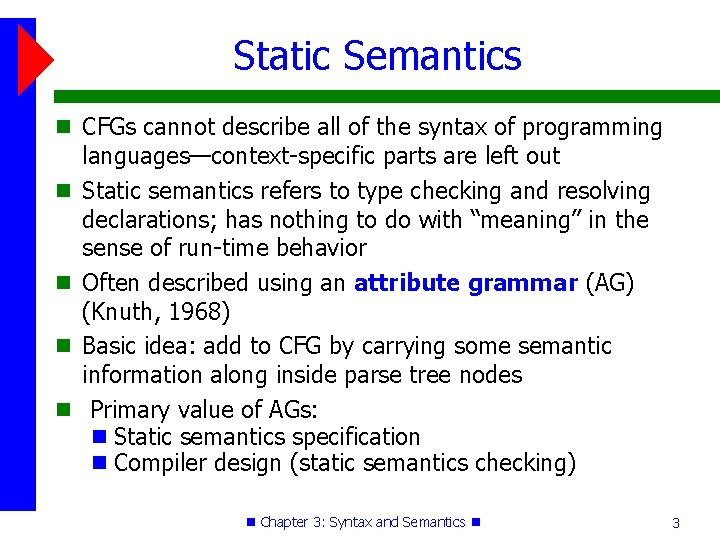Static Semantics CFGs cannot describe all of the syntax of programming languages—context-specific parts are left out Static semantics refers to type checking and resolving declarations; has nothing to do with “meaning” in the sense of run-time behavior Often described using an attribute grammar (AG) (Knuth, 1968) Basic idea: add to CFG by carrying some semantic information along inside parse tree nodes Primary value of AGs: Static semantics specification Compiler design (static semantics checking) Chapter 3: Syntax and Semantics 3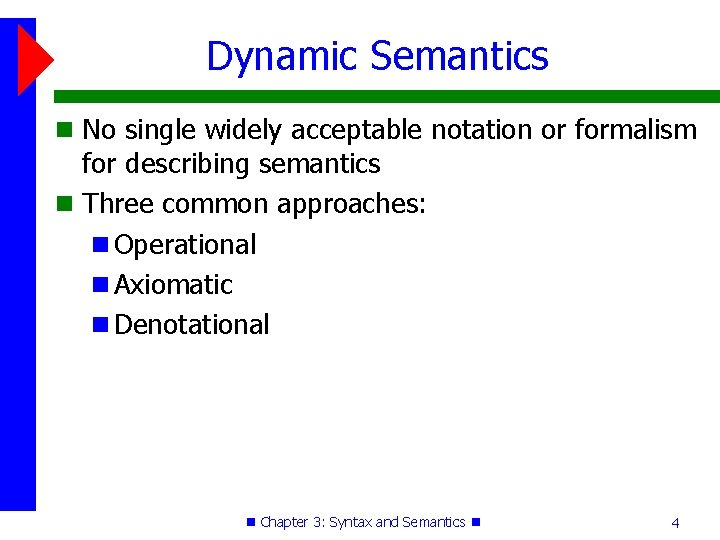Dynamic Semantics No single widely acceptable notation or formalism for describing semantics Three common approaches: Operational Axiomatic Denotational Chapter 3: Syntax and Semantics 4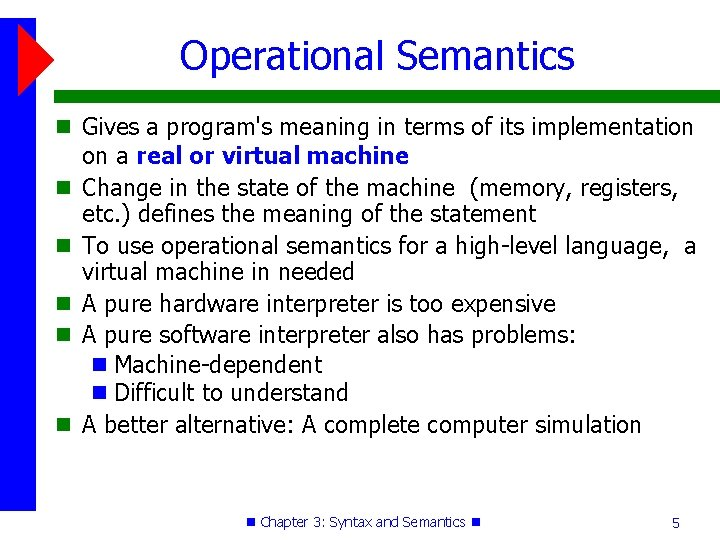Operational Semantics Gives a program's meaning in terms of its implementation on a real or virtual machine Change in the state of the machine (memory, registers, etc. ) defines the meaning of the statement To use operational semantics for a high-level language, a virtual machine in needed A pure hardware interpreter is too expensive A pure software interpreter also has problems: Machine-dependent Difficult to understand A better alternative: A complete computer simulation Chapter 3: Syntax and Semantics 5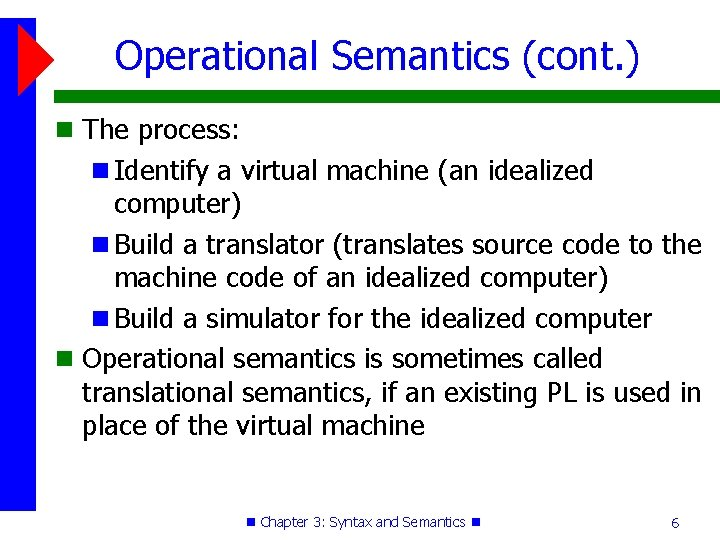Operational Semantics (cont. ) The process: Identify a virtual machine (an idealized computer) Build a translator (translates source code to the machine code of an idealized computer) Build a simulator for the idealized computer Operational semantics is sometimes called translational semantics, if an existing PL is used in place of the virtual machine Chapter 3: Syntax and Semantics 6Operational Semantics Example Pascal Operational Semantics for i : = x to y do begin. . . end i : = x loop: if i>y goto out. . . i : = i + 1 goto loop out: . . . Operational semantics could be much lower level: out: mov i, r 1 mov y, r 2 jmpifless(r 2, r 1, out). . . Chapter 3: Syntax and Semantics 7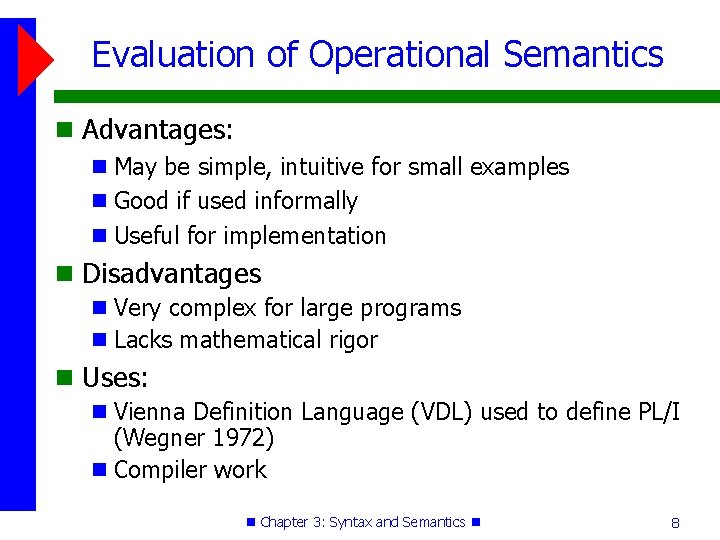Evaluation of Operational Semantics Advantages: May be simple, intuitive for small examples Good if used informally Useful for implementation Disadvantages Very complex for large programs Lacks mathematical rigor Uses: Vienna Definition Language (VDL) used to define PL/I (Wegner 1972) Compiler work Chapter 3: Syntax and Semantics 8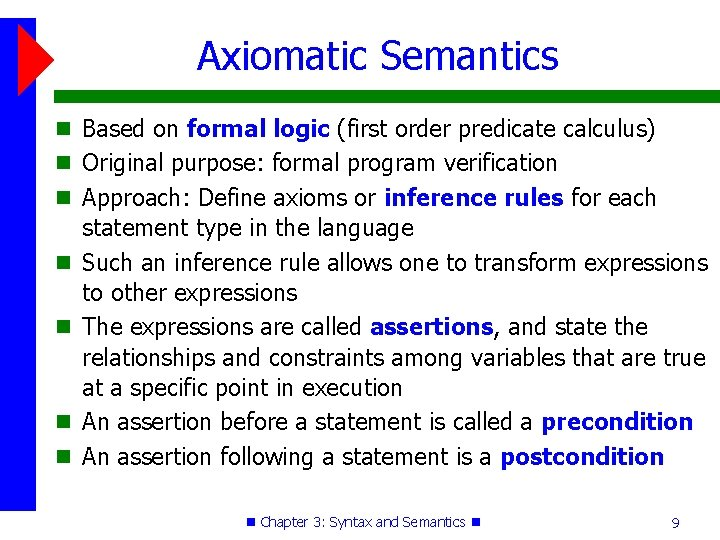Axiomatic Semantics Based on formal logic (first order predicate calculus) Original purpose: formal program verification Approach: Define axioms or inference rules for each statement type in the language Such an inference rule allows one to transform expressions to other expressions The expressions are called assertions, and state the relationships and constraints among variables that are true at a specific point in execution An assertion before a statement is called a precondition An assertion following a statement is a postcondition Chapter 3: Syntax and Semantics 9Weakest Preconditions Pre-post form: {P} statement {Q} A weakest precondition is the least restrictive precondition that will guarantee the postcondition An example: a : = b + 1 {a > 1} One possible precondition: {b > 10} Weakest precondition: {b > 0 } Chapter 3: Syntax and Semantics 10Program Proofs Program proof process: The postcondition for the whole program is the desired results Work back through the program to the first statement If the precondition on the first statement is the same as the program spec, the program is correct Chapter 3: Syntax and Semantics 11An Axiom for Assignment • An axiom for assignment statements: {Qx->E} x : = E {Q} P • Substitute E for every x in Q { P? } x : = y+1 { x > 0 } P = x > 0 x -> y+1 P = y+1 > 0 P = y > -1 • Basically, “undoing” the assignment and solving for y Chapter 3: Syntax and Semantics 12Some Inference Rules The Rule of Consequence: {P} S {Q}, P' => P, Q => Q' {P'} S {Q'} Antecedent implication consequent For a sequence S 1; S 2 the inference rule is: {P 1} S 1 {P 2}, {P 2} S 2 {P 3} {P 1} S 1; S 2 {P 3} Chapter 3: Syntax and Semantics 13A Rule for Loops An inference rule for logical pretest loops: {P} while B do S end {Q} The inference rule is: {I and B} S {I} while B do S {I and (not B)} Where I is the loop invariant. Chapter 3: Syntax and Semantics 14Loop Invariant Characteristicss I must meet the following conditions: 1. P I (the loop invariant must be true initially) 2. {I} B {I} (evaluation of the Boolean must not change the validity of I) 3. {I and B} S {I} (I is not changed by executing the body of the loop) 4. (I and (not B)) Q Q is implied) (if I is true and B is false, 5. The loop terminates (can be difficult to prove) Chapter 3: Syntax and Semantics 15More on Loop Invariants The loop invariant I is: A weakened version of the loop postcondition, and Also the loop’s precondition I must be: Weak enough to be satisfied prior to the beginning of the loop, but When combined with the loop exit condition, it must be strong enough to force the truth of the postcondition Chapter 3: Syntax and Semantics 16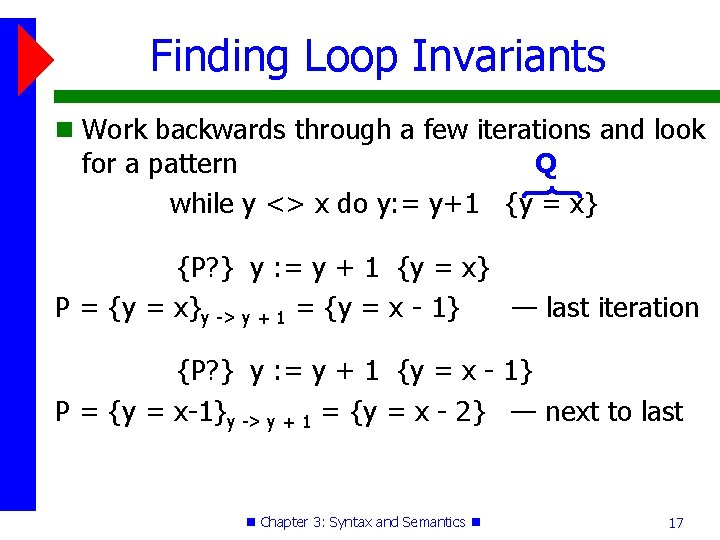Finding Loop Invariants Work backwards through a few iterations and look for a pattern Q while y <> x do y: = y+1 {y = x} {P? } y : = y + 1 {y = x} P = {y = x}y -> y + 1 = {y = x - 1} — last iteration {P? } y : = y + 1 {y = x - 1} P = {y = x-1}y -> y + 1 = {y = x - 2} — next to last Chapter 3: Syntax and Semantics 17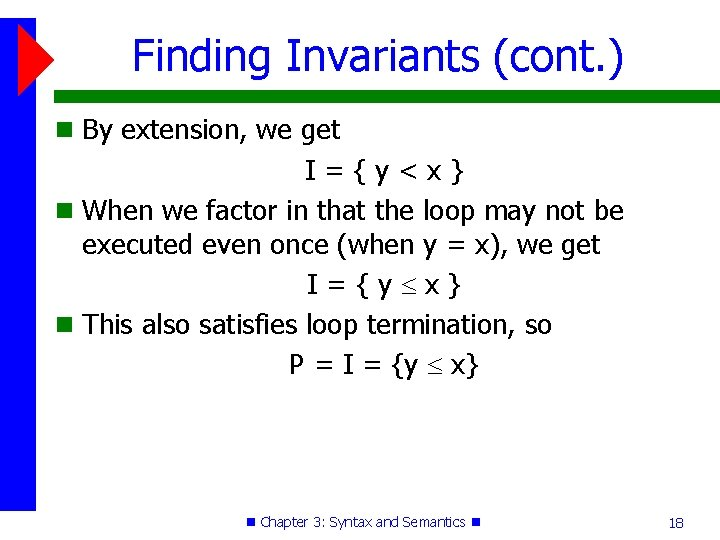Finding Invariants (cont. ) By extension, we get I={y<x} When we factor in that the loop may not be executed even once (when y = x), we get I={y x} This also satisfies loop termination, so P = I = {y x} Chapter 3: Syntax and Semantics 18Is I a Loop Invariant? (1) (2) (3) (4) (5) Does {y x} satisfy the 5 conditions? {y x} ? If {y x} and y <> x is then evaluated, is {y x} still true? If {y x} and y <> x are true and then y : = y+1 is executed, is {y x} true? Does {y x} and {y = x}? Can you argue convincingly that the program segment terminates? Chapter 3: Syntax and Semantics 19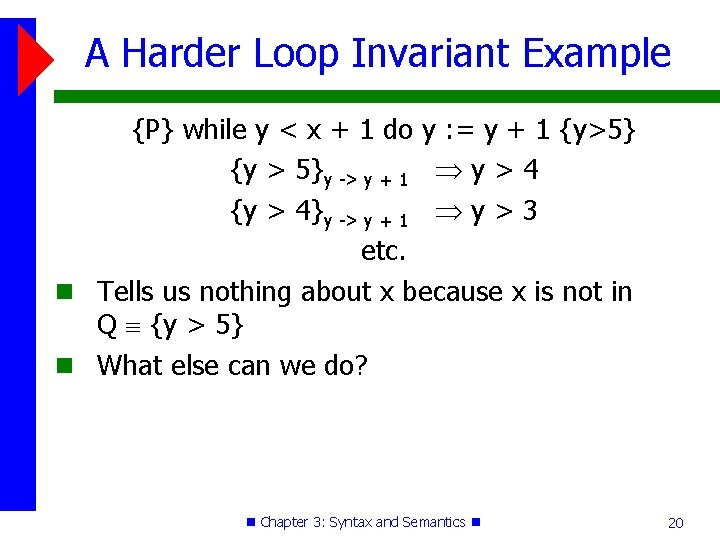A Harder Loop Invariant Example {P} while y < x + 1 do y : = y + 1 {y>5} {y > 5}y -> y + 1 y > 4 {y > 4}y -> y + 1 y > 3 etc. Tells us nothing about x because x is not in Q {y > 5} What else can we do? Chapter 3: Syntax and Semantics 20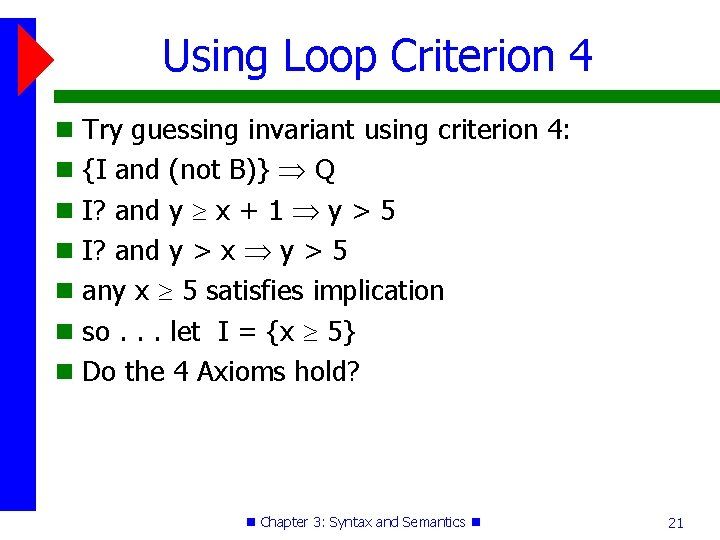Using Loop Criterion 4 Try guessing invariant using criterion 4: {I and (not B)} Q I? and y x + 1 y > 5 I? and y > x y > 5 any x 5 satisfies implication so. . . let I = {x 5} Do the 4 Axioms hold? Chapter 3: Syntax and Semantics 21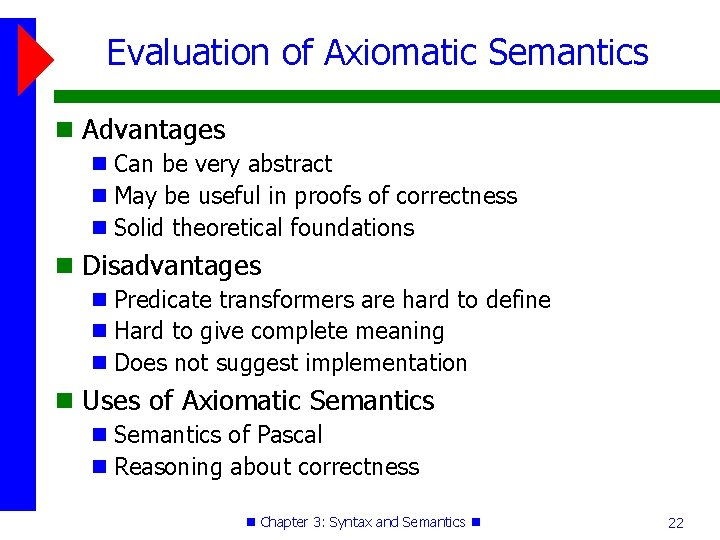Evaluation of Axiomatic Semantics Advantages Can be very abstract May be useful in proofs of correctness Solid theoretical foundations Disadvantages Predicate transformers are hard to define Hard to give complete meaning Does not suggest implementation Uses of Axiomatic Semantics of Pascal Reasoning about correctness Chapter 3: Syntax and Semantics 22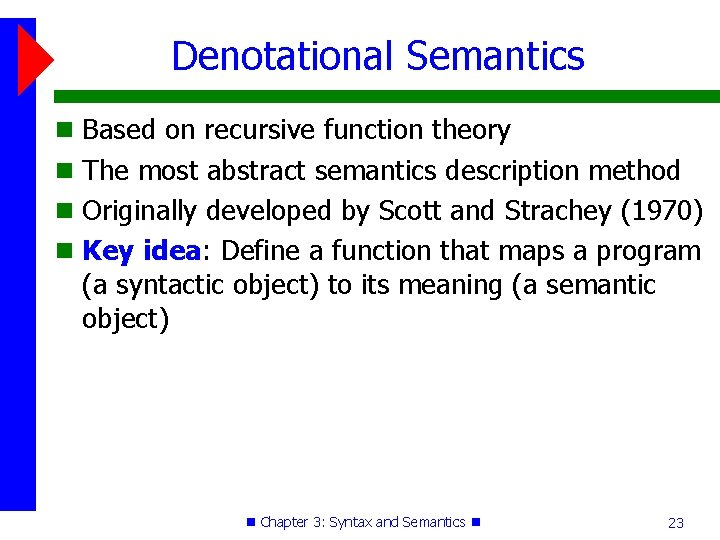Denotational Semantics Based on recursive function theory The most abstract semantics description method Originally developed by Scott and Strachey (1970) Key idea: Define a function that maps a program (a syntactic object) to its meaning (a semantic object) Chapter 3: Syntax and Semantics 23Denotational vs. Operational Denotational semantics is similar to high-level operational semantics, except: Machine is gone Language is mathematics (lamda calculus) The difference between denotational and operational semantics: In operational semantics, the state changes are defined by coded algorithms for a virtual machine In denotational semantics, they are defined by rigorous mathematical functions Chapter 3: Syntax and Semantics 24Denotational Specification Process 1. Define a mathematical object for each language entity 2. Define a function that maps instances of the language entities onto instances of the corresponding mathematical objects Chapter 3: Syntax and Semantics 25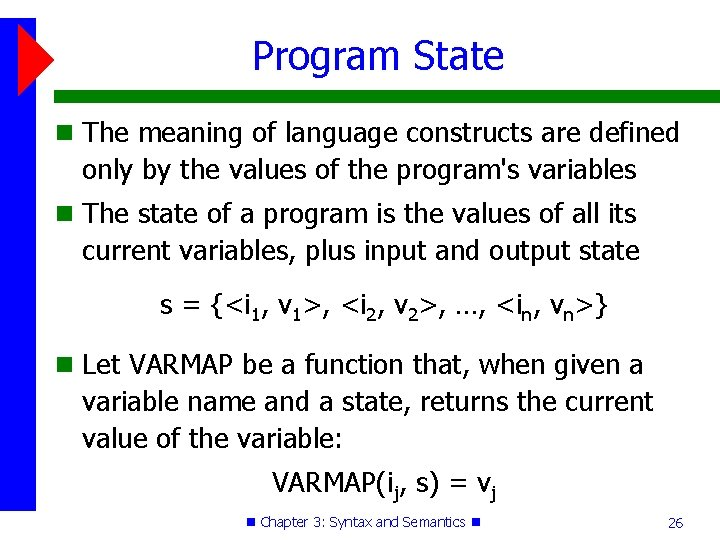Program State The meaning of language constructs are defined only by the values of the program's variables The state of a program is the values of all its current variables, plus input and output state s = {<i 1, v 1>, <i 2, v 2>, …, <in, vn>} Let VARMAP be a function that, when given a variable name and a state, returns the current value of the variable: VARMAP(ij, s) = vj Chapter 3: Syntax and Semantics 26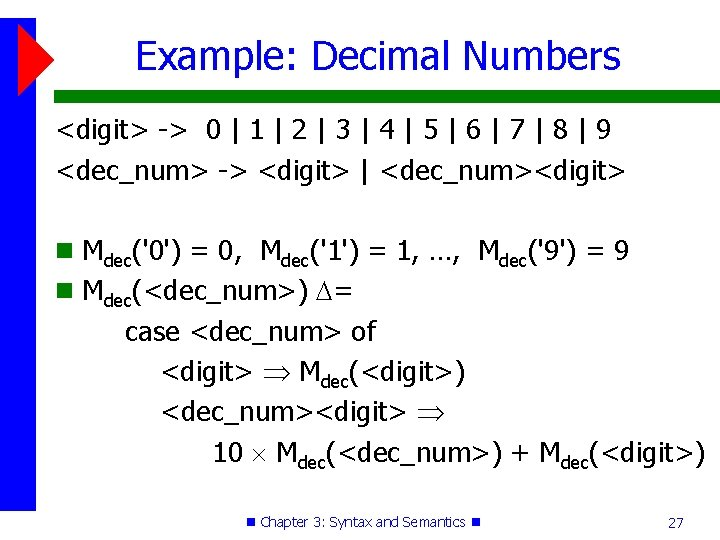Example: Decimal Numbers <digit> -> 0 | 1 | 2 | 3 | 4 | 5 | 6 | 7 | 8 | 9 <dec_num> -> <digit> | <dec_num><digit> Mdec('0') = 0, Mdec('1') = 1, …, Mdec('9') = 9 Mdec(<dec_num>) = case <dec_num> of <digit> Mdec(<digit>) <dec_num><digit> 10 Mdec(<dec_num>) + Mdec(<digit>) Chapter 3: Syntax and Semantics 27Expressions Me(<expr>, s) = case <expr> of <dec_num> Mdec(<dec_num>) <var> VARMAP(<var>, s) <binary_expr> if (<binary_expr>. <operator> = ‘+’) then Me(<binary_expr>. <left_expr>, s) + Me(<binary_expr>. <right_expr>, s) else Me(<binary_expr>. <left_expr>, s) Me(<binary_expr>. <right_expr>, s) Chapter 3: Syntax and Semantics 28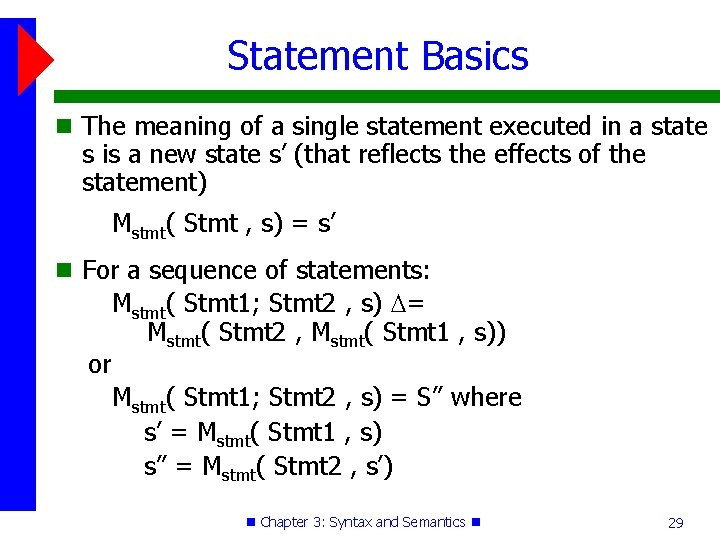Statement Basics The meaning of a single statement executed in a state s is a new state s’ (that reflects the effects of the statement) Mstmt( Stmt , s) = s’ For a sequence of statements: or Mstmt( Stmt 1; Stmt 2 , s) = Mstmt( Stmt 2 , Mstmt( Stmt 1 , s)) Mstmt( Stmt 1; Stmt 2 , s) = S’’ where s’ = Mstmt( Stmt 1 , s) s’’ = Mstmt( Stmt 2 , s’) Chapter 3: Syntax and Semantics 29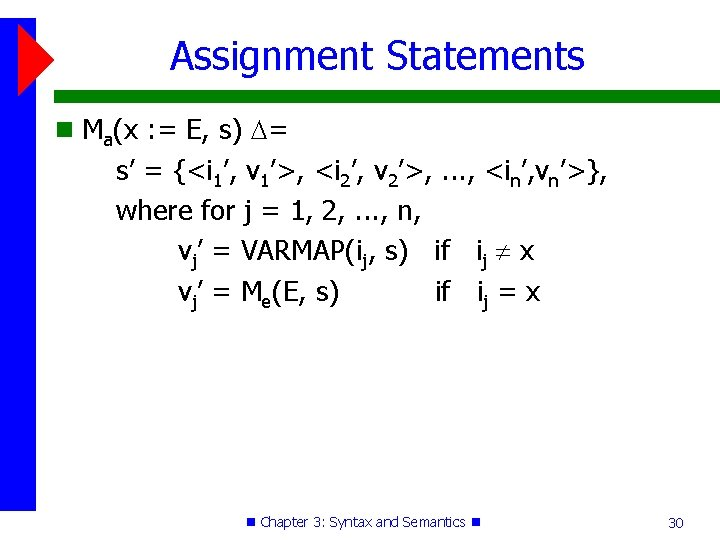Assignment Statements Ma(x : = E, s) = s’ = {<i 1’, v 1’>, <i 2’, v 2’>, . . . , <in’, vn’>}, where for j = 1, 2, . . . , n, vj’ = VARMAP(ij, s) if ij x vj’ = Me(E, s) if ij = x Chapter 3: Syntax and Semantics 30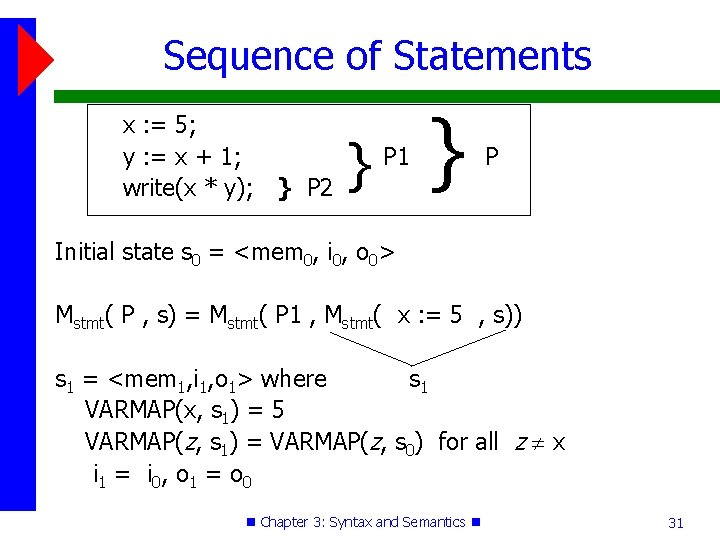Sequence of Statements x : = 5; y : = x + 1; write(x * y); } P 2 } P 1 } P Initial state s 0 = <mem 0, i 0, o 0> Mstmt( P , s) = Mstmt( P 1 , Mstmt( x : = 5 , s)) s 1 = <mem 1, i 1, o 1> where s 1 VARMAP(x, s 1) = 5 VARMAP(z, s 1) = VARMAP(z, s 0) for all z x i 1 = i 0, o 1 = o 0 Chapter 3: Syntax and Semantics 31Sequence of Statements (cont. ) Mstmt( P 1 , s 1) = Mstmt( P 2 , Mstmt( y : = x + 1 , s 1)) s 2 = <mem 2, i 2, o 2> where VARMAP(y, s 2) = Me( x + 1 , s 1) = 6 VARMAP(z, s 2) = VARMAP(z, s 1) for all z y i 2 = i 1 o 2 = o 1 Chapter 3: Syntax and Semantics 32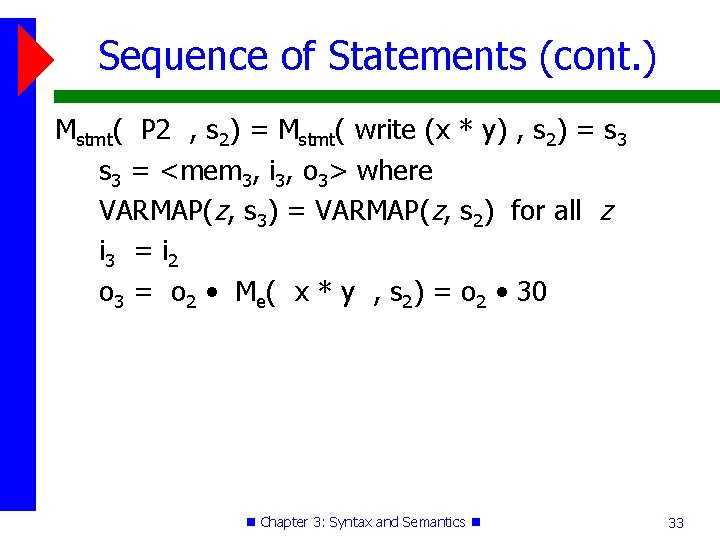Sequence of Statements (cont. ) Mstmt( P 2 , s 2) = Mstmt( write (x * y) , s 2) = s 3 = <mem 3, i 3, o 3> where VARMAP(z, s 3) = VARMAP(z, s 2) for all z i 3 = i 2 o 3 = o 2 • Me( x * y , s 2) = o 2 • 30 Chapter 3: Syntax and Semantics 33Sequence of Statements (concl. ) So, Mstmt( P , s 0) = s 3 = <mem 3, i 3, o 3 > where VARMAP(y, s 3) = 6 VARMAP(x, s 3) = 5 VARMAP(z, s 3) = VARMAP(z, s 0) for all z x, y i 3 = i 0 o 3 = o 0 • 30 Chapter 3: Syntax and Semantics 34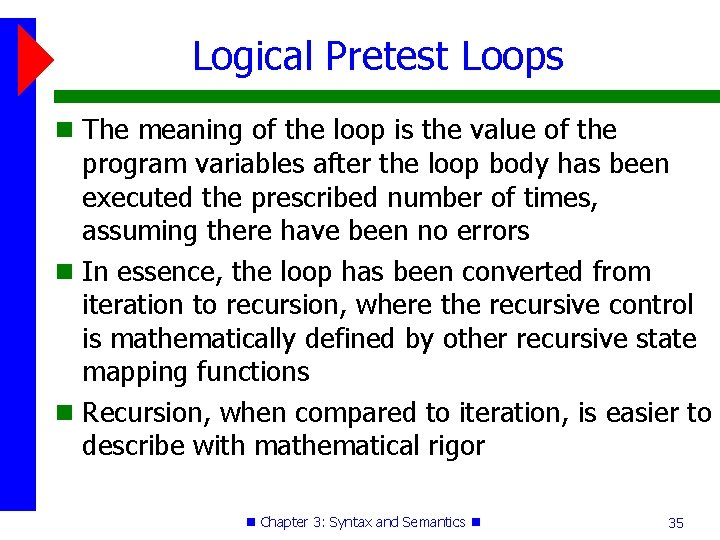Logical Pretest Loops The meaning of the loop is the value of the program variables after the loop body has been executed the prescribed number of times, assuming there have been no errors In essence, the loop has been converted from iteration to recursion, where the recursive control is mathematically defined by other recursive state mapping functions Recursion, when compared to iteration, is easier to describe with mathematical rigor Chapter 3: Syntax and Semantics 35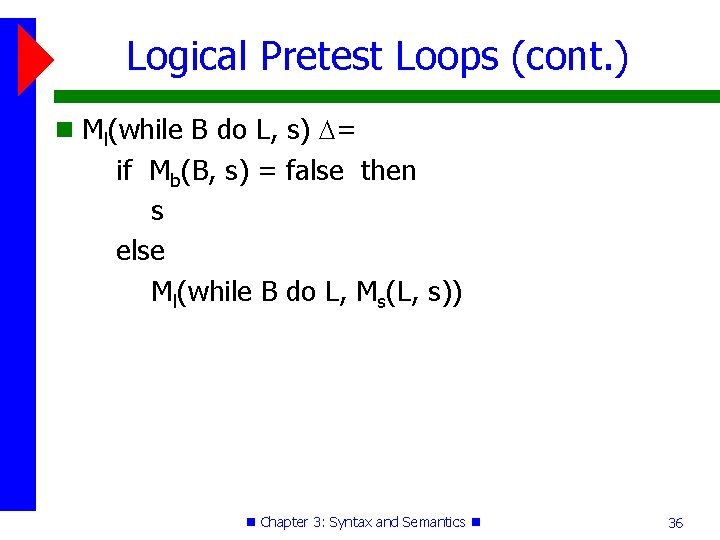Logical Pretest Loops (cont. ) Ml(while B do L, s) = if Mb(B, s) = false then s else Ml(while B do L, Ms(L, s)) Chapter 3: Syntax and Semantics 36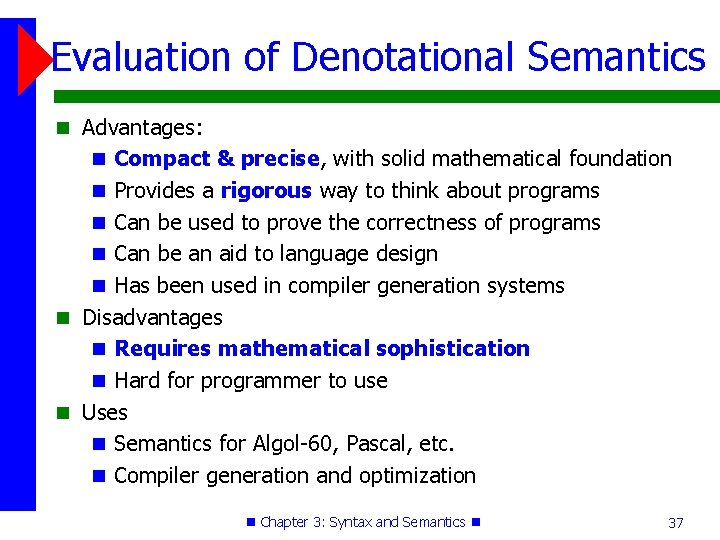Evaluation of Denotational Semantics Advantages: Compact & precise, with solid mathematical foundation Provides a rigorous way to think about programs Can be used to prove the correctness of programs Can be an aid to language design Has been used in compiler generation systems Disadvantages Requires mathematical sophistication Hard for programmer to use Uses Semantics for Algol-60, Pascal, etc. Compiler generation and optimization Chapter 3: Syntax and Semantics 37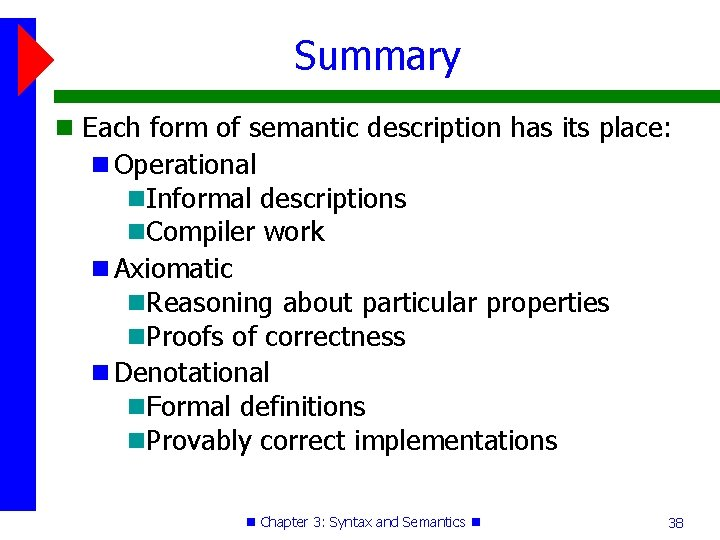Summary Each form of semantic description has its place: Operational Informal descriptions Compiler work Axiomatic Reasoning about particular properties Proofs of correctness Denotational Formal definitions Provably correct implementations Chapter 3: Syntax and Semantics 38